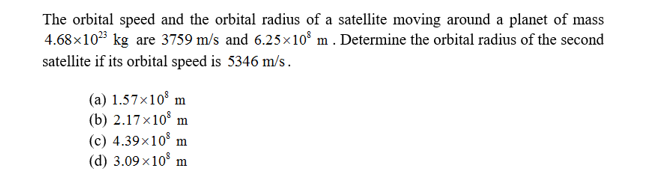# The orbital speed and the orbital radius of a satellite moving around a planet of mass4.68x1023 kg are 3759 m/s and 6.25x108 m . Determine the orbital radius of the secondsatellite if its orbital speed is 5346 m/s(a) 1.57x10 m(b) 2.17x10 m(c) 4.39x108 m(d) 3.09 x10

Question
99 viewshelp_outlineImage TranscriptioncloseThe orbital speed and the orbital radius of a satellite moving around a planet of mass 4.68x1023 kg are 3759 m/s and 6.25x108 m . Determine the orbital radius of the second satellite if its orbital speed is 5346 m/s (a) 1.57x10 m (b) 2.17x10 m (c) 4.39x108 m (d) 3.09 x10 fullscreen
check_circle

Step 1

The expression for the first satellite’s orbital speed is,

Step 2

Here, R1 is the first satellite’s orbital radius, G is the gravitational constant and M is the planet’s mass about which the satellite is orbiting.

The expression for the second satellite’s orbital speed is,

Step 3

Here, R2 is the second satellite’s orbital radius.

Take the rati...

### Want to see the full answer?

See Solution

#### Want to see this answer and more?

Solutions are written by subject experts who are available 24/7. Questions are typically answered within 1 hour.*

See Solution
*Response times may vary by subject and question.
Tagged in

### Gravitation